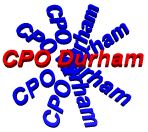How Much Chemical?

 FORMULA HMC = A x ( B1 ÷ B2 ) x (C1 ÷ C2)

 HMC = How much chemical A = Amount of chemical from the CPO Durham handout or book B1 = the size of your pool B2 = 40,000 L. C1 = Your desired changed C2 = The amount of change in ppm in 40,000 L.

EXAMPLE